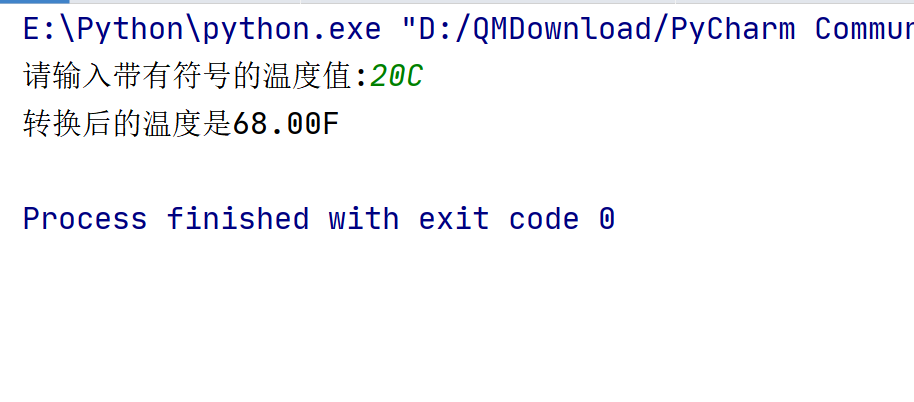• ## Python实现温度转换

千次阅读 2020-06-16 16:19:32
题目描述： 华氏度F和摄氏度C的转换公式为：F=C*1.8+32。 输入带有符号的温度值，格式为...实现代码： TempStr=input("请输入带有符号的温度值:") if TempStr[-1] in ['F','f']: try: C=(eval(TempStr[0:-1])-32)/1.8
题目描述：
华氏度F和摄氏度C的转换公式为：F=C*1.8+32。
输入带有符号的温度值，格式为：温度值加上单位。如果单位为C或c，则表示为摄氏度，将其转换为华氏度;如果单位为F或f，则表示为华氏度，将其转换为摄氏度。如果输入格式不正确，输出“输入格式错误”的提示信息。
实现代码：
TempStr=input("请输入带有符号的温度值:")
if TempStr[-1] in ['F','f']:
try:
C=(eval(TempStr[0:-1])-32)/1.8
print("转换后的温度是{:.2f}C".format(C))
except NameError:
print("输入格式错误")
elif TempStr[-1] in ['C','c']:
try:
F=1.8*eval(TempStr[0:-1])+32
print("转换后的温度是{:.2f}F".format(F))
except NameError:
print("输入格式错误")
else:
print("输入格式错误")

运行演示：展开全文算法
• 温度的刻画有两个不同体系：摄氏度（Celsius）和华氏（Fabrenheit）。‪‬‪‬‪‬‪‬‪‬‮‬‫‬‭‬‪‬‪‬‪‬‪‬‪‬‮‬‫‬‫‬‪‬‪‬‪‬‪‬‪‬‮‬‪‬‪‬‪‬‪‬‪‬‪‬‪‬‮‬‫‬‪‬‪‬‪...

温度的刻画有两个不同体系：摄氏度（Celsius）和华氏（Fabrenheit）。‪‬‪‬‪‬‪‬‪‬‮‬‫‬‭‬‪‬‪‬‪‬‪‬‪‬‮‬‫‬‫‬‪‬‪‬‪‬‪‬‪‬‮‬‪‬‪‬‪‬‪‬‪‬‪‬‪‬‮‬‫‬‪‬‪‬‪‬‪‬‪‬‪‬‮‬‭‬‪‬‪‬‪‬‪‬‪‬‪‬‮‬‪‬‭‬请编写程序将用户输入华氏度转换为摄氏度，或将输入的摄氏度转换为华氏度。‪‬‪‬‪‬‪‬‪‬‮‬‫‬‭‬‪‬‪‬‪‬‪‬‪‬‮‬‫‬‫‬‪‬‪‬‪‬‪‬‪‬‮‬‪‬‪‬‪‬‪‬‪‬‪‬‪‬‮‬‫‬‪‬‪‬‪‬‪‬‪‬‪‬‮‬‭‬‪‬‪‬‪‬‪‬‪‬‪‬‮‬‪‬‭‬转换算法如下：（C表示摄氏度、F表示华氏度）‪‬‪‬‪‬‪‬‪‬‮‬‫‬‭‬‪‬‪‬‪‬‪‬‪‬‮‬‫‬‫‬‪‬‪‬‪‬‪‬‪‬‮‬‪‬‪‬‪‬‪‬‪‬‪‬‪‬‮‬‫‬‪‬‪‬‪‬‪‬‪‬‪‬‮‬‭‬‪‬‪‬‪‬‪‬‪‬‪‬‮‬‪‬‭‬
C = ( F - 32 ) / 1.8
F = C * 1.8+ 3
代码：

temp=input()
if temp in ['F','f']:
c=(eval(temp[1:])-32)/1.8
print("C{:.2f}".format(c))
elif temp in ['C','c']:
f=eval(temp[1:])*1.8+32
print("F{:.2f}".format(f))
else:
print("erro")




展开全文• 主要介绍了python实现简单温度转换的方法,涉及Python操作字符串的技巧,具有一定参考借鉴价值,需要的朋友可以参考下
•temp
• #实现功能----温度转换 ''' 温度单位 （1） 摄氏度c/C （2） 华氏度f/F 摄氏温度c/C ,将其转化为华氏温度f /F, 转换公式为：f=c*9/5+32. 华氏温度f/F,将其转换为摄氏温度c/C c=(f-32)/1.8 ''' TempStr = input("请...
#实现功能----温度转换
'''
温度单位 （1） 摄氏度c/C （2） 华氏度f/F
摄氏温度c/C ,将其转化为华氏温度f /F,
转换公式为：f=c*9/5+32.
华氏温度f/F,将其转换为摄氏温度c/C
c=(f-32)/1.8
'''

TempStr = input("请输入带符号的温度：")
if TempStr[-1] in ['F','f'] :
C = (eval(TempStr[0:-1]) - 32)/1.8
print("转换后的温度为{:.2f}C".format(C))
elif TempStr[-1] in  ['C','c'] :
F = 1.8*eval(TempStr[0:-1]) + 32
print("转换后的温度为{:.2f}F" .format(F))
else:
print("温度输入错误")

'''
学习内容
缩进 【代表层次关系】注释【说明】 命名【数字不开头】 变量 保留字【标识符】
数据类型 字符串 整数 浮点数 列表
赋值语句 分支语句 函数
input() print() eval()
'''


展开全文• 实现温度转换为华氏温度实现代码如下： Tempstr=input(请输入需要转换的温度值:) if Tempstr[-1] in ['F','f']: C=(eval(Tempstr[0:-1])-32)/1.8 print(转换后的温度值为 {:.2f}C.format(C
• 主要介绍了Python实现制度转换（货币，温度，长度），文中通过示例代码介绍的非常详细，对大家的学习或者工作具有一定的参考学习价值，需要的朋友们下面随着小编来一起学习学习吧
• # @Author : 望天宇八方清似玉 # @CreateTime: 2019/4/22 17:50 # @File : Temp.py ...TempStr = input("请输入带有符号的温度值: ") if TempStr[-1] in ['F', 'f']: C =(eval(TempStr[0:-1])-32)/1.8...
# @Author    : 望天宇八方清似玉
# @CreateTime: 2019/4/22 17:50
# @File      : Temp.py
# @Software  : PyCharm
TempStr = input("请输入带有符号的温度值: ")
if TempStr[-1] in ['F', 'f']:
C =(eval(TempStr[0:-1])-32)/1.8
print("转换后的温度是:{:.2f}C".format(C))
elif TempStr[-1] in ['C', 'c']:
F = 1.8*eval(TempStr[0:-1])+32
print("转换后的温度是:{:.2f}F".format(F))
else:print("你输入的格式有误")


如果不能运行：

1.切换到python3版本

2.头部指定编码方式

展开全文• 利用python实现简单的华氏温度和摄氏温度转换，采用python实现
• 今天学习了python实现温度转换的案例，代码如下 TempStr = input("请输入带有符号的温度值：") if TempStr[-1] in ['F','f']: C = (eval(TempStr[0:-1]) - 32)/1.8 print("转换后的温度是{:.2f}C".format(C)) elif...
• TempStr=input("请输入带有符号的温度值，C/c表示摄氏度... print("转换后的温度是{:.2f}C".format(C)) elif TempStr[-1] in ['C','c']: F=1.8*eval(TempStr[0:-1])+32 print("转换后的温度是{:.2f}F".format(F)) el.编程语言
• t=input() if t[-1] in ['F','f']: c=(eval(t[0:-1])-32)/1.8 print('{:.2f}C'.format(c)) elif t[-1] in ['C','c']: f=eval(t[0:-1])*1.8+32 print('{:.2f}F'.format(f)) else: print('输入格式错误')
• 利用Python实现的华氏度与摄氏度之间的转换，可以很好作为Python入门实例
• 人民币和美元是世界上通用的两种货币之一，写一个程序进行货币间币值转换，其中：人民币和美元间汇率固定为：1美元 = 6.78人民币。程序可以接受人民币或美元输入，转换为美元或人民币输出。人民币采用RMB表示，美元...
• 实现温度转换：1、C = （F-32）/1.8 2、F = C*1.8 + 32TempStr = input("请输入带有温度单位(C\F)的值：(回车退出)")while TempStr !="": if TempStr[-1] in ['C','c']: F = eval(TempStr[:-...
• python tkinter库实现华氏温度摄氏温度转换功能要求创建GUI窗口创建文本标签创建可输入文本按钮控件创建显示文本框温度转换函数-g总程序结束啦 功能要求 创建一个图形窗口，其中有一个是输入框，用于输入摄氏温度，...tkinter
• 本文主要研究的是Python语言实现温度转换的相关实例，具体如下。 代码如下： #TempConvert.py val=input("请输入带有温度表示符号的温度值（例如：32c）") if val[-1] in ["C","c"]: f=1.8*float(val[0:-1])+32 ...
• 1.根据输入内容判断单位类型2.完成温度互转，华氏温度与摄氏温度，3.完成长度互转，中国与美国长度单位4...content = {'T':'温度转换', 'L':'长度转换', 'D':'货币转换' } for k,v in content.items(): print(k,...
• Python写程序实现摄氏温度与华氏温度转换 F=input('请输入华氏温度：'); C=(float(F)-32)/1.8; print('对应的摄氏温度：',C);
• 实现温度转换类型标识符在尾部的转换，例如12F、12f、12C和12c等，其中F|f表示华氏温度，C|c表示设施温度 TempStr = input("请输入符号在数字后的温度值:") if TempStr[-1] in ['F','f']: C = (eval(TempStr[0:-1])...
• 用户输入对应工具的数字（1/2/3/4）调用工具（BMR计算/汇率转换/温度转换），根据工具的提示输入参数，获得相应输出。同时，用户的相关操作会保存到文件中。 二 代码实现如下 """ 作者：zhengzhihui 版本：...生活小工具 bmr计算 汇率转换
• 本文主要通过一段简单代码，介绍在python实现温度转换的一个样例。 代码来自mooc国家精品课程《Python语言程序设计》，也是我重温python的指导课程。 我在每行代码后都做了备注，方便大家进行理解。 ''' 规定输入...
• #tempconvert.py def tempConvert(ValueStr): if ValueStr[-1] in ['F','f']: C=(eval(ValueStr[0:-1])-32)/1.8 print("转换后的温度是{:.2f}C".format(C)) elif ValueStr[-1] in ['C','c']: ...# python实现温度转换python 订阅